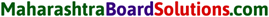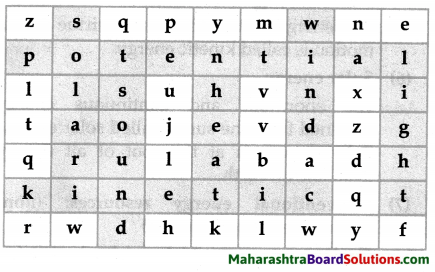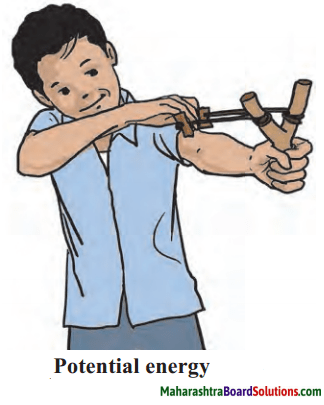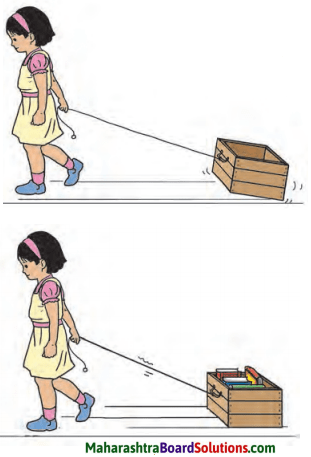# Maharashtra Board Class 6 Science Solutions Chapter 11 Work and Energy

Balbharti Maharashtra State Board Class 6 Science Solutions Chapter 11 Work and Energy Notes, Textbook Exercise Important Questions and Answers.

## Maharashtra State Board Class 6 Science Solutions Chapter 11 Work and Energy

Class 6 Science Chapter 11 Work and Energy Textbook Questions and Answers

1. Fill in each blank with the appropriate term from the brackets.

Question a.
A bucketful of water is to be drawn from a well ………. will be done when a ………… is applied to do this, because there will be an ……………. of water.
(displacement, workforce)
Answer:
work, force, displacement

Question b.
If a ball is dropped on the sloping roof of a house, it acquires ……………. and falls on the ground. That is transformation of ………… energy into ……….. energy takes place.
(kinetic, potential, motion)
Answer:
motion, kinetic, potentialQuestion c.
You might have seen some beautiful fireworks during Diwali. It is an example of transformation of ……….. energy into ………………. energy.
(light, atom, chemical, solar)
Answer:
chemical, light

Question d.
The solar cooker is an application of the ………… energy of the sun, while solar cells, solar lamps are applications of the ………….. energy of the sun.
(light, chemical, heat)
Answer:
heat, light

Question e.
One labourer carried four pans of road metal through a distance of 100 metres. If he carries two pans of road metal through a 200 metre distance …………. work will be done.
(equal, more, less)
Answer:
equal

Question f.
The capacity that an object has for doing work is called ………… .
(energy, displacement, force)
Answer:
energy2. Match the pairs.

Question a.

 Column ‘A’ Column ‘B’ 1. Rolling object a. Heat energy 2. Food b. Atomic energy 3. Stretched bow c. Kinetic energy 4. Sunlight d. Potential energy 5. Uranium e. Chemical energy

Answer:

 Column ‘A’ Column ‘B’ 1. Rolling object c. Kinetic energy 2. Food e. Chemical energy 3. Stretched bow d. Potential energy 4. Sunlight a. Heat energy 5. Uranium b. Atomic energy3. Can you tell?

Question a.
When can we say that displacement has taken place?
Answer:
When the position of an object changes, we say that the object is displaced.

Question b.
What are the various forms of energy?
Answer:
Various forms of energy are: mechanical energy, heat energy, light energy, sound energy, chemical energy and electrical energy.Question c.
Describe the natural chain of transformation of energy.
Answer:

1. In the course of water cycle, water evaporates due to the heat of the sun.
2. This water vapour forms clouds that give rain.
3. Rainwater flows into rivers and is stored in reservoirs or dams.
4. This stored water at a height has potential energy.
5. As the water falls down, this is transformed into kinetic energy.
6. When the water falls on the turbine, its kinetic energy is transferred to turbine.
7. The turbine rotates producing electrical energy.

Question d.
What should be taken into account for measuring work?
Answer:
For measuring work both force and displacement must be taken into account.

Question e.
Why should we save energy?
Answer:
Due to increasing population and increasing use of energy resources, there is a danger that limited reserves of coal, petrol, etc. will get exhausted. Hence, we should save energy for future generation.Question f.
What is ‘green energy’?
Answer:
The energy resources which do not produce smoke and carbon gases such as carbon dioxide or carbon monoxide are called ‘green energy resources’. Energy from them is called green energy.

Question g.
What are the non-conventional energy resources?
Answer:
The resources that:

1. have not been used traditionally.
2. are inexhaustible and continuous.
3. That can be used in various forms again and again are called non-conventional energy resources.
4. e.g.: solar energy, wind energy, tidal energy, hydal power.

Question h.
Which forms of energy from the sun is used in solar energy devices?
Answer:

1. Heat energy from the sun is used in solar cookers, solar water heaters, solar driers.
2. Light energy from the sun is used in solar cells, solar panels.
3. Solar electric plants have the capacity to produce electricity on large scale.Question i.
Why should we maximize the use of non- ntional energy sources?
Answer:

1. Due to increase in population there is increasing use of conventional sources like petrol, coal, etc. Their reserves are limited and they may get exhausted.
2. Hence, it will be better to use non- conventional sources of energy to save conventional resources for future.

4. Who is the odd-one-out?

Class 6 Science Chapter 11 Work And Energy Question 1.
Diesel, crude oil, natural gas, wind.
Answer:
wind

Work And Energy Class 6 Maharashtra Board Question 2.
A running car, hauling a log, a book kept on a table, picking up the school bag.
Answer:
A book kept on table.

Work And Energy Class 6 Question 3.
Sunlight, wind, wave, petrol.
Answer:
Petrol.11 Work And Energy Class 6 Question 4.
Leaving the fan on in a vacant room, leaving the TV on while working, using A.C during winter, putting off the light when going out.
Answer:
putting off the light when going out.

5. Find out the types of energy from the following puzzle.

Question a.
Find out the types of energy from the following puzzle.Answer:
Potential, Kinetic, Solar, Wind, Sound, Light, HeatActivity:

Work And Energy Exercise Class 6 Question 1.
How can you save energy at home and in school? Discuss this with your friends and list all such measures. Put them into practice.Work And Energy Class 6 Questions Answers Question 2.
Find out about energy saving devices available in the market.

Class 6 Science Chapter 11 Work and Energy Important Questions and Answers

Fill in the blanks with suitable words.

Work And Energy Class 6 Exercise Question 1.
Falling water possesses ………………… energy.
(potential, kinetic, chemical)
Answer:
kinetic

Work And Energy Class 6 Questions And Answers Question 2.
Heat is measured in unit called ……………….. .
(kilometres, kilogram, calorie)
Answer:
calorie

Class 6 Science Chapter 11 Question 3.
Plant stores the food in the form of ……………….. energy.
(mechanical, light, chemical)
Answer:
chemical11. Work And Energy Class 6 Question 4.
We should use ……………….. energy resources with care.
(conventional, non-conventional, both)
Answer:
conventional

Match the columns:

Question 1.

 Column ‘A’ Column ‘B’ 1. Solar energy a. Waves 2. Tidal energy b. Home cooking 3. Natural gas c. Car 4. Petrol d. Solar water heater

Answer:

 Column ‘A’ Column ‘B’ 1. Solar energy d. Solar water heater 2. Tidal energy a. Waves 3. Natural gas b. Home cooking 4. Petrol c. Car

Can you tell?

6th Science Work And Energy Question 1.
Water in dams or reservoirs shows potential energy.
Answer:
Rainwater flows into river and is stored in reservoirs on dams. These are at height and therefore, their water has potential energy.Class 6 Science Chapter 11 Question Answer Question 2.
The sun is the most important source of all energy.
Answer:
All forms of energy are utilizing indirectly solar energy in all transformation of energy. Therefore, the sun is the most important source of all energy.

Question 3.
What is tidal energy?
Answer:
A wall is constructed at a narrow region of a creek. Due to waves generated by high and low tides, the turbine of the generator fitted in the wall starts rotating and electricity is generated.

Use your brain power!

Question 1.
Why do you get hungry after physical exercise?
Answer:

1. When we do physical exercise we use the energy stored in the body.
2. To replenish this energy, we have to eat. Hence we feel hungry.

Question 2.
From where does our body get energy.
Answer:
Our body gets energy from the food we eat.

Question 3.
Why do we get tired?
Answer:
When we do any work, we use the energy. If this energy level becomes very low, then we feel tired.Question 4.
What is the unit of work and energy?
Answer:
The unit of work and energy in SI (System International) is ‘Joule’.

Question 5.
If no displacement occurs on applying a force, what does it indicate?
Answer:
If no displacement occurs, then no work is said to be performed.

Can you tell?

Question 1.
In the game of marbles, from where does one marble get energy to set another marble in motion?
Answer:
When we apply physical force to the fast marble, it gains some force and when it strikes the second marble, energy in the form of force is transferred to the second marble and this force sets the second marble in motion.

Question 2.
While playing carrom from where does the striker gets the energy to make the pieces move?
Answer:
When we strike the striker, the force exerted by our muscles is transferred to the striker. When the striker strikes the coin, this energy is transferred to the coin which may help to pocket the coin.Question 3.
What will happen as result of the action in the picture?Question i.
The rubber band is released after streaching it.
Answer:
It comes back to its orriginal position.

Question ii.
A stone is held in the rubber of a catapult, the rubber is stretched and released.
Answer:
Stone gets thrown to a distance and rubber band cones back to its original position.

Let’s try this.

Question 1.
If we hold a convex lens over a sheet of paper in sunlight such that there is tiny spot of light on the paper for a while. What happens? Why?
Answer:

1. If a convex lens is held over a paper in sunlight such that there is a tiny spot of light on the paper for a while the paper bums.
2. This is due to the heat energy of the sun concentrated at a point due to the convex lens.Question 2.
How does a picture appear on the screen of a TV?
Answer:
When a TV is switched on, the electrical energy is converted into light and sound energy. Hence, a picture appears on TV.

Question 3.
In which form is energy stored in plant food?
Answer:
Energy stored in plant food is in the form of chemical energy.

Question 4.
How is energy obtained from cooking gas?
Answer:
Energy obtained from cooking gas is heat energy which is obtained by conversion of chemical energy.

Question 5.
Why is Energy not visible to us?
Answer:
Energy is the capacity to do work. Energy is converted from one form to another in the form of work being done. Hence it is not visible to us.

How are we different from each other?

Question 1.
Potential energy and Kinetic energy
Answer:

 Potential energy Kinetic energy 1. Energy stored in an object due to its position is called potential energy. 1. Energy possessed by a body due to its motion is called kinetic energy. 2. No work is performed. 2. Work is performed.Question 2.
Work and energy
Answer:

 Work Energy 1. When an object is displaced by applying force on it, work is said to be done. 1. Energy is the capacity to do work. 2. Work is done as a result of using energy. 2. Energy is the cause for work to be done.

Question 3.
Conventional and Non-conventional energy sources
Answer:

 Conventional source of energy Non-Conventional source of energy 1. Man has been using these energy resources for centuries. 1. These resources have not been used traditionally.

Classify as directed:

Question 1.
Into kinetic and potential energy
Waterfalls, water in a reservoir, a stretched spring, a boy running in a race.
Answer:

• Potential – water in a reservoir, a stretched spring.
• Kinetic – waterfalls, a boy running in a race.Question 2.
Into conventional and non-conventional energy sources.
Tidal energy, diesel, cooking gas, coal, hydropower, solar energy.
Answer:

• Conventional energy sources – diesel, cooking gas, coal.
• Non-conventional energy sources – tidal energy, hydropower, solar energy.

Question 3.
Into different forms of energy

1. rubber band is released
2. cooking food
3. switching on fan
4. a battery
5. radio
6. TV

Answer:

1. Mechanical energy
2. Heat energy
3. Mechanical energy, electrical energy
4. Chemical energy
5. Sound energy
6. Light and sound energyDefine:

Question 1.
Work
Answer:
When an object is displaced by applying a force on it, work is said to be done.

Question 2.
Energy
Answer:
Energy is the capacity to do work.

Question 3.
Chemical energy
Answer:
The energy obtained through chemical action is called chemical energy.Question 4.
Potential energy
Answer:
The energy which is stored in an object due to a specific state or position of that object is called potential energy.

Question 5.
Kinetic energy
Answer:
The energy obtained by the virtue of its motion is called kinetic energy.

Question 6.
Solar energy
Answer:
The enormous and continuous energy obtained from the sun is called solar energy. Solar energy is at the root of all energy available on earth.

Question 7.
Conventional energy resources: (non-renewable energy sources)
Answer:
The energy sources which man has been using for centuries and that cannot be replenished are called conventional energy sources.

Question 8.
Renewable energy sources: (non – conventional energy sources)
Answer:
The resources that have not been used traditionally and that are inexhaustible, continuous and can be used in various forms again and again are called renewable energy sources.

Explain – why?

Question 1.
Energy is not visible to us.
Answer:
There is energy in every substance in the universe. It is present in non-living as well as living things. But still we can only feel the energy when some work is done, e.g. When wind blows, we can feel the coolness in air. When we switch on the light in a dark room, we can see things due to light energy.Question 2.
Save energy, (use alternative energy sources)
Answer:

1. Due to increasing population, there is increasing usage of energy resources.
2. There is a danger that limited reserves of conventional resources like diesel, crude oil, may get exhausted.
3. We should save energy so that future availability is ensured.
4. It will be better to use alternative and supplementary energy sources.

Question 3.
Methods of saving energy.
Answer:

1. Saving energy is as good as generating energy.
2. There are several ways of saving energy such as putting off lamps when not needed.
3. Making maximum use of sunlight.
4. Saving energy and energy resources is absolutely necessary. Otherwise, we will have to face the serious calamity like global warming.

Question 4.
State the energy conversions in following cases.

1. Solar water heater
2. Solar cell
3. Fission of uranium, thorium
4. Switch on mixer
5. Switch on lamp
6. Switch on TV
7. Switch on radio
8. Ram plays tabla
9. Ravi plays shehnai
10. You switch on the torch
11. Your mother lights the gas stove to cook
12. Dinesh is bursting crackers during Diwali

Answer:

1. Solar energy to heat energy
2. Solar energy to electrical energy
3. Atomic energy to heat energy and then to electrical energy
4. Electrical energy to mechanical energy
5. Electrical energy to light energy
6. Electrical energy to light energy and sound energy
7. Electrical energy to sound energy,
8. Mechanical energy to sound energy
9. Wind energy to sound energy
10. Chemical energy to light energy
11. Chemical energy to heat energy
12. Chemical energy to heat, light and sound energyObserve the figure below and answer the question given.Question i.
In the above activity, which are the different forces acting on the box?
Answer:
In the above activity, muscular force, frictional force and gravitational force act on the box.

Question ii.
Is displacement possible without force?
Answer:
No, force has to be applied in order to displace the box.

Question iii.
If force is applied to both the boxes is same, which box will be displaced more?
Answer:
If force applied to both the boxes is same, then empty box will be displaced more than box with books.Question iv.
If me e books are added to box, and same force is applied, will the displacement be more or less?
Answer:
If the same force is applied for a heavier load then the displacement will be less.

Question v.a.
If a force is applied with both the hands on a wall, will there be a displacement?
Answer:
Whatever be the amount of force applied, the wall will not be displaced.

Question b.
If no displacement occurs on applying as force, what does it indicate?
Answer:
If no displacement occurs, then no work is said to be performed.

Scroll to Top